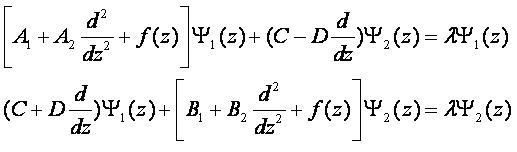# finite difference method

## Finite Difference Method Boundary conditions at corner

Choose a channel featured in the header of iMechanica:

I am implementing finite difference scheme for the simple 2D Laplace
equation. I am facing problem in imposing boundary conditions in 9-point
stencil. What boundary conditions should I give at the vertex i.e. at
the corner where two boundaries intersect.

e.g.- For square plate what should be boundary condition at four vertices?

What should be the boundary condition at vertices in Dirichlet problem?either homogeneous or non-homogeneous?

## Find eigenvalues of the coupled differential equations by Finite Difference Method.

Hello everybody,

The coupled differential equations need to solve as following:Could you help me to discretize this systems and find the eigenvalue λ  numerically using finite difference method?

lqkhai